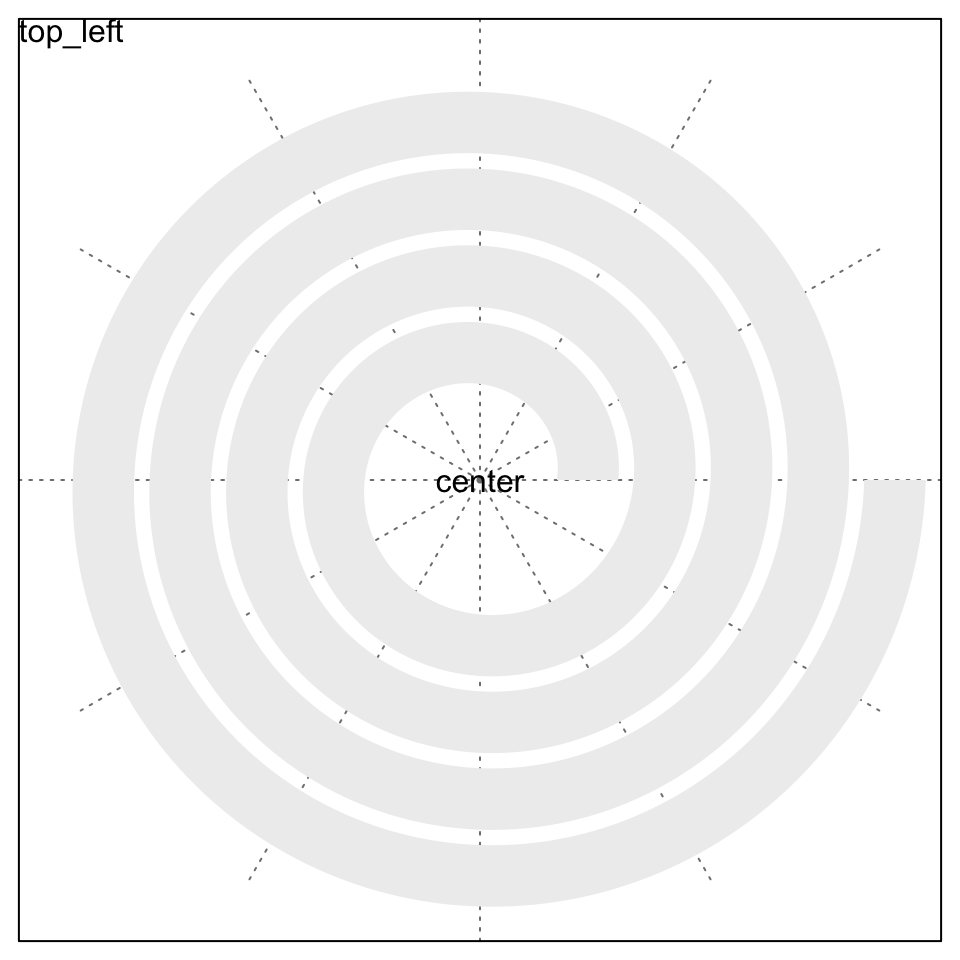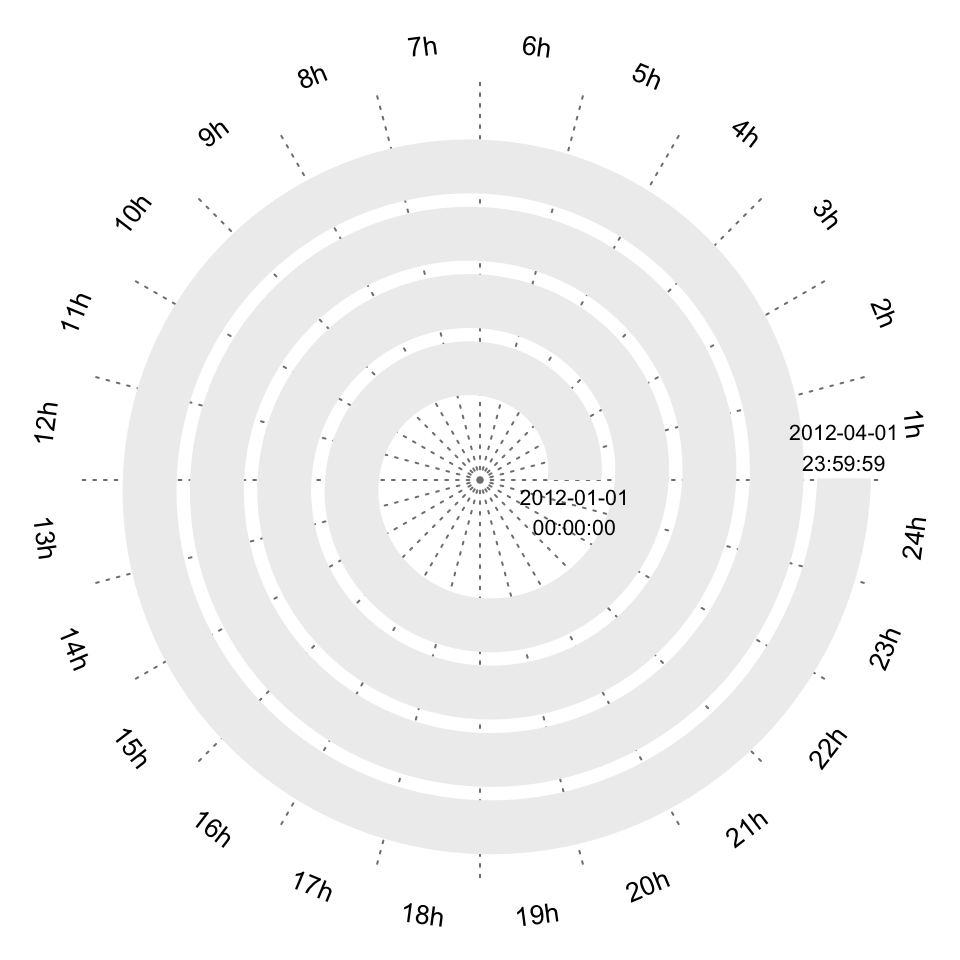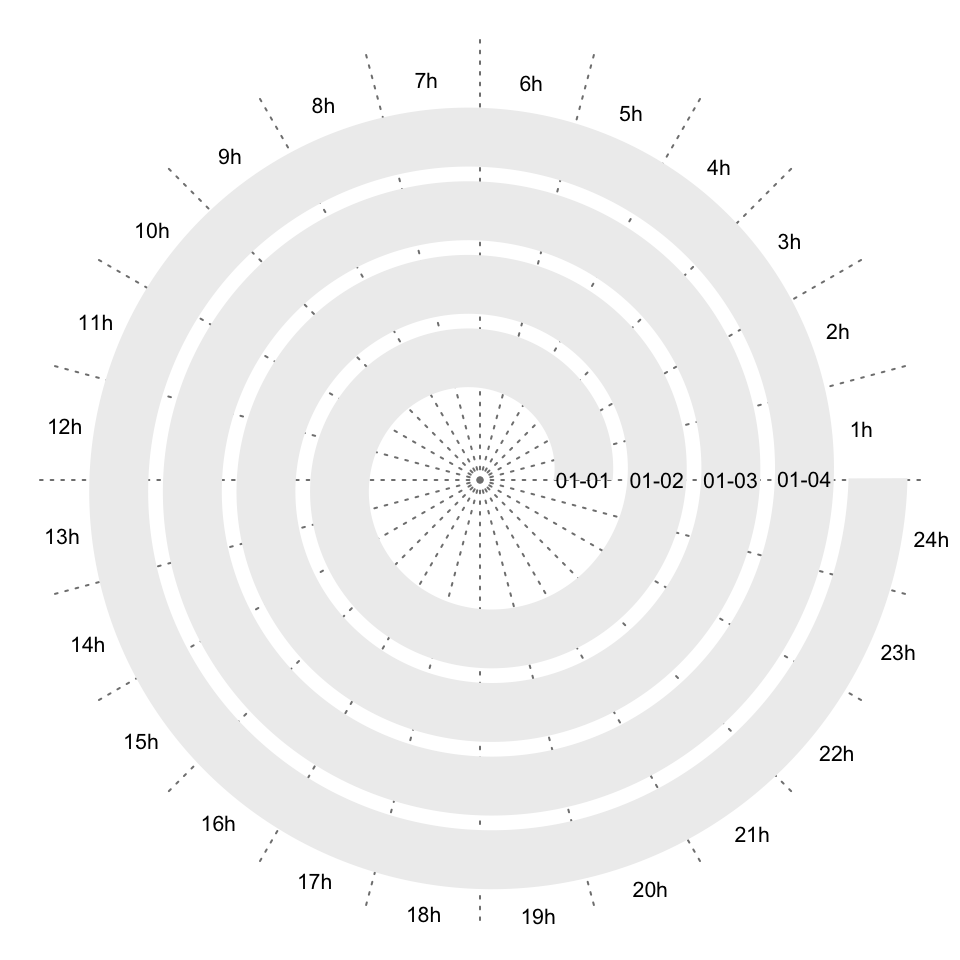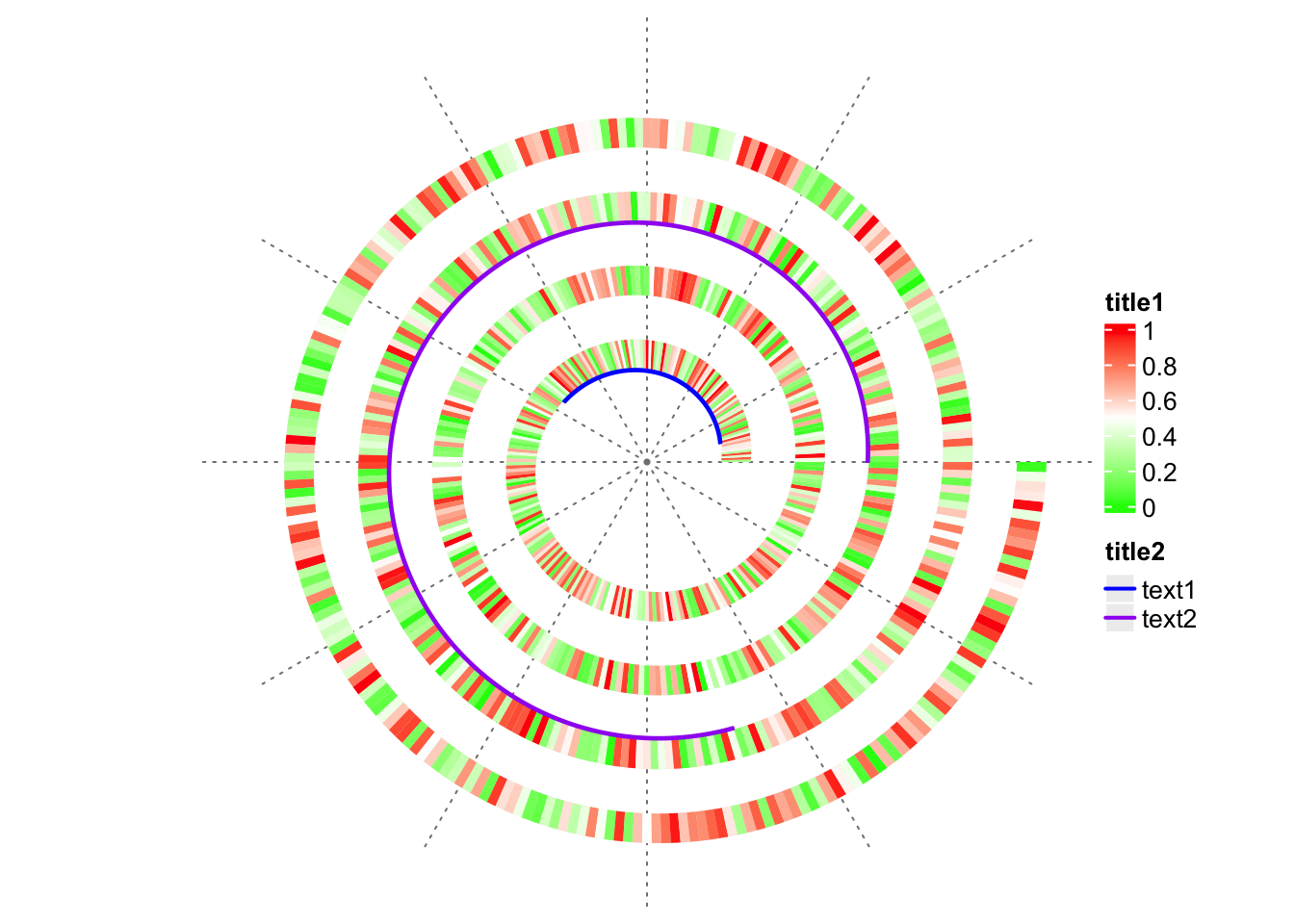# Annotate the Plot

#### 2022-02-04

This vignette is built with spiralize 1.0.5.

In this vignette, I demonstrate how to add text and legend to the spiral plot.

## Text

spiralize is implemented under grid graphics system, thus functions from grid need to be used with spiralize.

The spiral plot is drawn in a square viewport, as shown in the following figure, thus, simply you can use `grid.text()` to add text to a specific position.

``````library(spiralize)
spiral_initialize()
spiral_track()
grid.rect(gp = gpar(fill = NA))
grid.text("center", 0, 0, default.units = "native")
grid.text("top_left", 0, 1, default.units = "npc", just = c("left", "top"))``````For time series data, users might want to add texts to describe different “seasons/sectors”. In the following example, assume period is day, I add hour names to the corresponding sectors in the polar coordinate system. I also add text to the start and end of the spiral.

``spiral_initialize_by_time(xlim = c("2021-01-01 00:00:00", "2021-01-04 23:59:59"), padding = unit(2, "cm"))``
``````## 'unit_to_axis' is set to 'mins'.
## 'period' is set to 'days'.``````
``````spiral_track()

s = current_spiral()

d = seq(360/24/2, 360, by = 360/24) %% 360
for(i in seq_along(d)) {
df = polar_to_cartesian(d[i]/180*pi, (s\$max_radius + 1.5))
grid.text(paste0(i, "h"), x = df[1, 1], y = df[1, 2], default.unit = "native",
rot = ifelse(d[i] > 0 & d[i] < 180, d[i] - 90, d[i] + 90), gp = gpar(fontsize = 10))
}

x = s\$get_x_from_data(c("2021-01-01 00:00:00", "2021-01-04 23:59:59"))
df = xy_to_cartesian(x, 0.5)
grid.text("2012-01-01\n00:00:00", df\$x, df\$y, default.units = "native", vjust = 1.2,
gp = gpar(fontsize = 8))
grid.text("2012-04-01\n23:59:59", df\$x, df\$y, default.units = "native", vjust = -0.2,
gp = gpar(fontsize = 8))``````The drawback of the previous plot is e.g. 1h and 2h are too far away to the spiral. Users might want to put the text closer to the spiral. This can be done by just drawing text in the track, but with y-locations larger than the maximal y-location in the track (drawing above the plot region of the track).

``spiral_initialize_by_time(xlim = c("2021-01-01 00:00:00", "2021-01-04 23:59:59"), padding = unit(1, "cm"))``
``````## 'unit_to_axis' is set to 'mins'.
## 'period' is set to 'days'.``````
``````spiral_track()

# ymax of the track is 1, but here we draw the text at y = 1.5
library(lubridate)
spiral_text(as.POSIXlt("2021-01-04 00:30:00") + lubridate::hours(0:23), y = 1.5, paste0(1:24, "h"), gp = gpar(fontsize = 8))
spiral_text("2021-01-01 00:00:00", 0.5, "01-01", gp = gpar(fontsize = 8))
spiral_text("2021-01-02 00:00:00", 0.5, "01-02", gp = gpar(fontsize = 8))
spiral_text("2021-01-03 00:00:00", 0.5, "01-03", gp = gpar(fontsize = 8))
spiral_text("2021-01-04 00:00:00", 0.5, "01-04", gp = gpar(fontsize = 8))``````## Legend

Legends are important graphics elements in a plot which guild users to understand the plot. spiralize does not directly draw legends, but it can be very easily done with other legend functions, such as `Legend()` and `packLegend()` from ComplexHeatmap package.

`Legend()` creates a single legend, either continuous or discrete, and `packLegend()` combines a list of legends into a single object. Check the following example:

``````n = 1000
spiral_initialize(xlim = c(0, n))
spiral_track(height = 0.4)

x = runif(n)
col_fun = circlize::colorRamp2(c(0, 0.5, 1), c("green", "white", "red"))
spiral_rect(1:n - 1, 0, 1:n, 1, gp = gpar(fill = col_fun(x), col = NA))

spiral_highlight(10, 100, type = "line", gp = gpar(col = "blue"))
spiral_highlight(500, 700, type = "line", gp = gpar(col = "purple"))

library(ComplexHeatmap)
lgd = packLegend(
Legend(title = "title1", col_fun = col_fun),
Legend(title = "title2", type = "lines", legend_gp = gpar(col = c("blue", "purple"), lwd = 2),
at = c("text1", "text2"))
)
draw(lgd, x = unit(1, "npc") + unit(2, "mm"), just = "left")``````When I draw the legend, I put the legend to a position of `unit(1, "npc") + unit(2, "mm")` which is 2mm to the right of the spiral plot. To make the legend properly visible, you must set the image width larger than the image height in order to give space to the legend (in the image above, the width is 7 inch and the height is 5 inch).

If you also want to add title for the plot, you need to do a little bit more. One simple way is to push several viewports, as shown in the folowing code.

``````grid.newpage()
title_height = grobHeight(textGrob("A", gp = gpar(fontsize = 14)))*2
pushViewport(viewport(y = 1, height = title_height, just = "top"))
grid.text("This is a title", gp = gpar(fontsize = 14))
popViewport()

pushViewport(viewport(y = 0, height = unit(1, "npc") - title_height, just = "bottom"))
spiral_initialize(xlim = c(0, n), newpage = FALSE)  # here setting newpage = FALSE is important!
spiral_track(height = 0.4)
spiral_rect(1:n - 1, 0, 1:n, 1, gp = gpar(fill = col_fun(x), col = NA))

spiral_highlight(10, 100, type = "line", gp = gpar(col = "blue"))
spiral_highlight(500, 700, type = "line", gp = gpar(col = "purple"))

draw(lgd, x = unit(1, "npc") + unit(2, "mm"), just = "left")

spiral_clear()
popViewport()``````